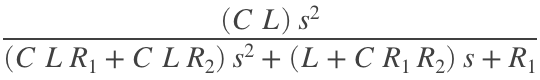## 1. Laplace Transform

Perform a Laplace transform of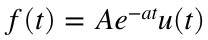% Tell matlab what variables we'll be using
syms A a t
% Use function laplace() to get the Laplace transform
laplace(A*exp(-a*t))
ans =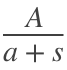The final answer is## 2. Inverse Laplace Transform

Find the inverse Laplace transform of% Tell matlab what variables we'll be using
syms s
% Use function ilplace() to get the inverse Laplace transform
ilaplace(1/(s+3)^2)
ans =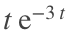The final answer is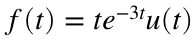## 3. Partial Fraction Expansion

### Case 1: Roots of the denominator are real and distinct

Perform a partial-fraction expansion of% Define the numerator polynomial coefficients
num = 2;
% Define the denominator polynomial coefficients
% The conv() function will multiply (s+1)(s+2) to
% get the coefficients of the polynomial
denom = conv([1 1],[1 2]);
% Use residue function to get residues and poles
[r,p] = residue(num,denom)
r = 2×1
-2 2
p = 2×1
-2 -1
We see that the residuals are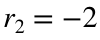and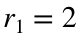and that the poles are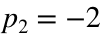and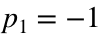. The expanded partial fraction looks like this:### Case 2: Roots of the denominator are real and repeated

Perform a partial fraction expansion of% Define numerator
num = 2;
% Define denominator
denom = conv([1 1],conv([1 2], [1 2]));
% Use residue function to get residues and poles
[r,p] = residue(num,denom)
r = 3×1
-2.0000 -2.0000 2.0000
p = 3×1
-2.0000 -2.0000 -1.0000
The residues are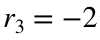,, and. The poles are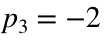,and. The expanded partial fraction is:### Case 3: Roots of the denominator are complex or imaginary

Expand the fraction% Define numerator
num = 3;
% Define denominator
denom = conv([1 0],[1 2 5]);
% Use residue function
[r,p]=residue(num,denom)
r = 3×1 complex
-0.3000 + 0.1500i -0.3000 - 0.1500i 0.6000 + 0.0000i
p = 3×1 complex
-1.0000 + 2.0000i -1.0000 - 2.0000i 0.0000 + 0.0000i
This expansion has three residues and three poles: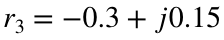,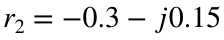, and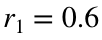. Poles are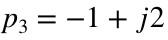,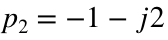, and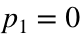. The expanded partial fraction looks like:## 4. Miscellaneous Matlab Operations

### Multiply polynomials together

Multiply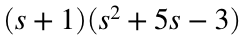% Use conv() to multiply polynomials together
% The coefficients go in an array []
% So (s+1) looks like [1 1]
% and (s^2+5s-3) looks like [1 5 -3]
conv([1 1],[1 5 -3])
ans = 1×4
1 6 2 -3
The final polynomial is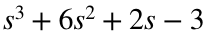### Find the roots of a polynomial

Find the roots of% Use the roots() function to find the roots of a polynomial
% given an array of the coefficients
roots([1 6 2 -3])
ans = 3×1
-5.5414 -1.0000 0.5414
The roots are equal to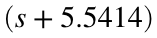,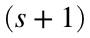, and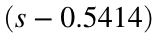.

### Solve a set of simultaneous equations

Solve the following set of equations for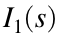and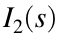.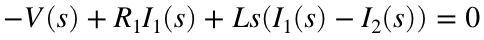% Define symbols
syms s R1 R2 L C I1 I2 V
% Enter each equation on a line
eqn1 = -V + R1*I1 + L*s*(I1-I2) == 0;
eqn2 = L*s*(I2-I1) + R2*I2 + I2/(C*s) == 0;
% Use Matlab to solve the equations for I1 and I2
[solI1,solI2]=solve([eqn1,eqn2],[I1,I2])
solI1 =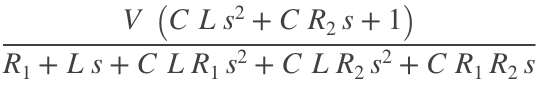solI2 =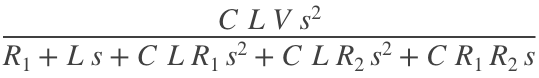These are the answers forand. To get the transfer functionwe do this
% The transfer func G = I2(s)/V(s) is
G = solI2/V;
% To make it pretty, we can use collect() to group the coefficients of the
% polynomial before printing
collect(G,s)
ans =